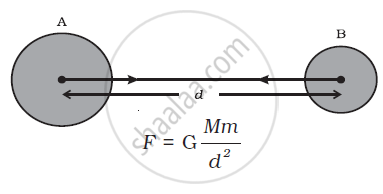Advertisement Remove all ads

# Universal Law of Gravitation

Advertisement Remove all ads

#### notes

Universal Law of Gravitation

Every object in the universe attracts other object by a force of attraction, called gravitation, which is directly proportional to the product of masses of the objects and inversely proportional to the square of distance between them. This is called Law of Gravitation or Universal Law of Gravitation.

Let masses (M) and (m) of two objects are distance (d) apart. Let F be the attractional force between two masses.According to the universal law of gravitation, the force between two objects is directly proportional to the product of their masses. That is,

F∝M × m                             ....(1)

And the force between two objects is inversely proportional to the square of the distance between them, that is,

F ∝ 1/d                              ....(2)

Combining Eqs. (1) and (2), we get,

"F" ∝ ("M" xx "m") / "d"^2

or "F" = "G" ("M" xx "m")/"d"^2           ...(3)

where G is the constant of proportionality and is called the universal gravitation constant. By multiplying crosswise, Eq. (3) gives

F × d 2 = G M × m

or "G" = ("F""d"^2) / ("M" xx "m")      ...(4)

The SI unit of G can be obtained by substituting the units of force, distance and mass in Eq.(4) as N m2 kg–2. The value of G was found out by Henry Cavendish (1731 – 1810) by using a sensitive balance. The accepted value of G is 6.673 × 10–11 N m2 kg–2.

If you would like to contribute notes or other learning material, please submit them using the button below.

### Shaalaa.com

Universal law of gravitation [00:38:58]
S
##### Series:
0%

Advertisement Remove all ads
Share
Notifications

View all notifications

Forgot password?